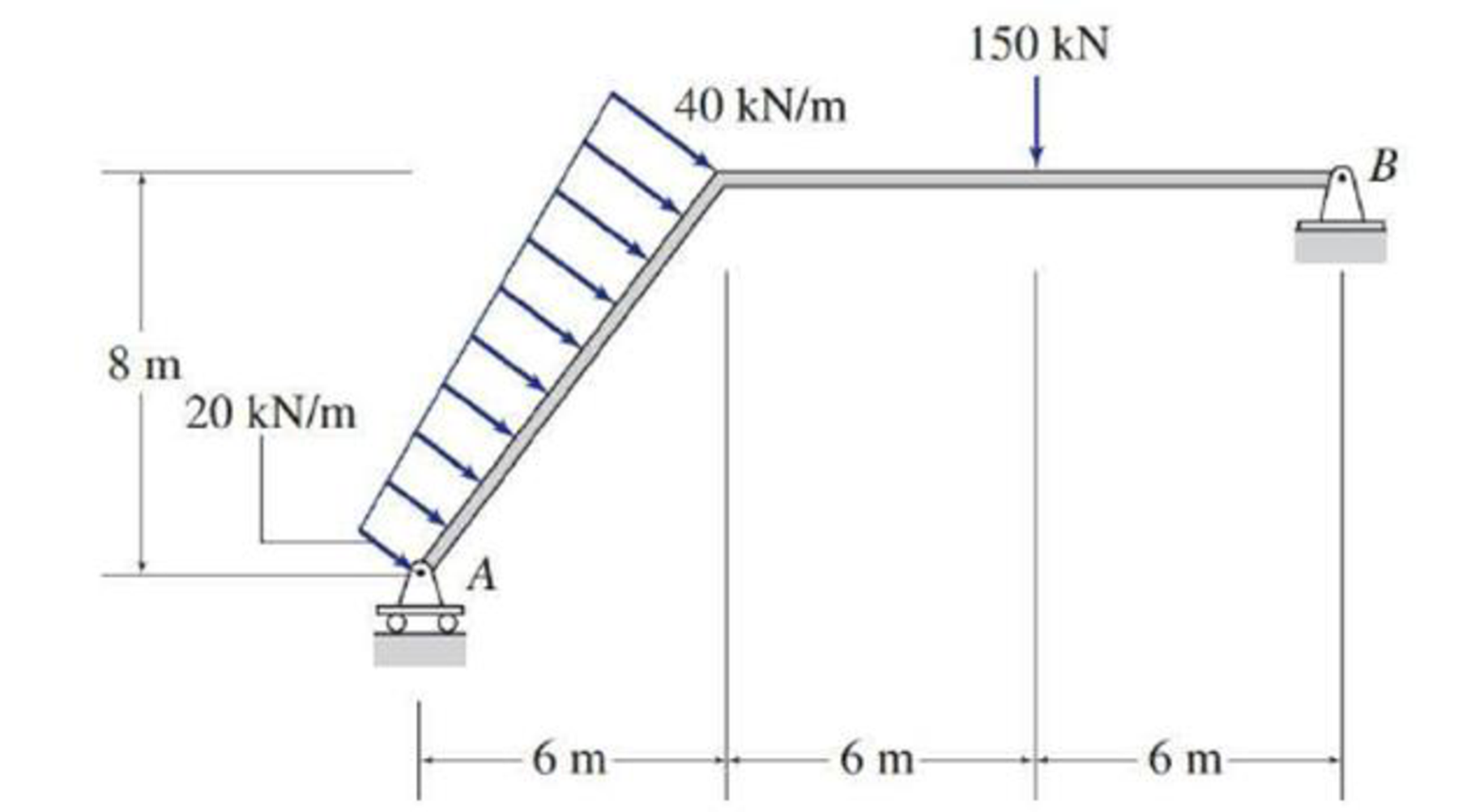# Determine the reactions at the supports for the structures shown. FIG.P3.23

#### Solutions

Chapter
Section
Chapter 3, Problem 23P
Textbook Problem
90 views

## Determine the reactions at the supports for the structures shown.FIG.P3.23

To determine

Calculate the support reactions for the given structure.

### Explanation of Solution

Given information:

The structure is given in the Figure.

Apply the sign conventions for calculating reaction forces and moments using the three equations of equilibrium as shown below.

• For summation of forces along x-direction is equal to zero (Fx=0), consider the forces acting towards right side as positive (+) and the forces acting towards left side as negative ().
• For summation of forces along y-direction is equal to zero (Fy=0), consider the upward force as positive (+) and the downward force as negative ().
• For summation of moment about a point is equal to zero (Matapoint=0), consider the clockwise moment as negative and the counter clockwise moment as positive.

Calculation:

Let Bx and By be the horizontal and vertical reactions at the hinged support B.

Let Ay be the vertical reaction at the roller support A.

Sketch the free body diagram of the beam as shown in Figure 1.

Use equilibrium equations:

Summation of forces along x-direction is equal to 0.

+Fx=0Bx+(20+402)(10)(45)=0Bx+240=0Bx=240kN

Therefore, the horizontal reaction at B is Bx=240kN_.

Summation of moments about A is equal to 0

### Still sussing out bartleby?

Check out a sample textbook solution.

See a sample solution

#### The Solution to Your Study Problems

Bartleby provides explanations to thousands of textbook problems written by our experts, many with advanced degrees!

Get Started

Find more solutions based on key concepts
Draw the top, the front and the right side orthogonal views of the given object and indicate when an object nee...

Engineering Fundamentals: An Introduction to Engineering (MindTap Course List)

Participate in an Online Auction Online auctions allow consumers to bid on products that other people are selli...

Enhanced Discovering Computers 2017 (Shelly Cashman Series) (MindTap Course List)

Describe the type(s) of relationship(s) between STORE and REGION.

Database Systems: Design, Implementation, & Management

What does AISI stand for?

Precision Machining Technology (MindTap Course List)

Describe modular design, and explain the two main prototyping methods.

Systems Analysis and Design (Shelly Cashman Series) (MindTap Course List)

What is a subtype discriminator? Give an example of its use.

Database Systems: Design, Implementation, & Management

Determine the axial force in member BC of the frame. Note the joint D is a sliding pin.

International Edition---engineering Mechanics: Statics, 4th Edition

If your motherboard supports ECC DDR3 memory, can you substitute non-ECC DDR3 memory?

A+ Guide to Hardware (Standalone Book) (MindTap Course List)

What do the two pressure gauges on a regulator show?

Welding: Principles and Applications (MindTap Course List)